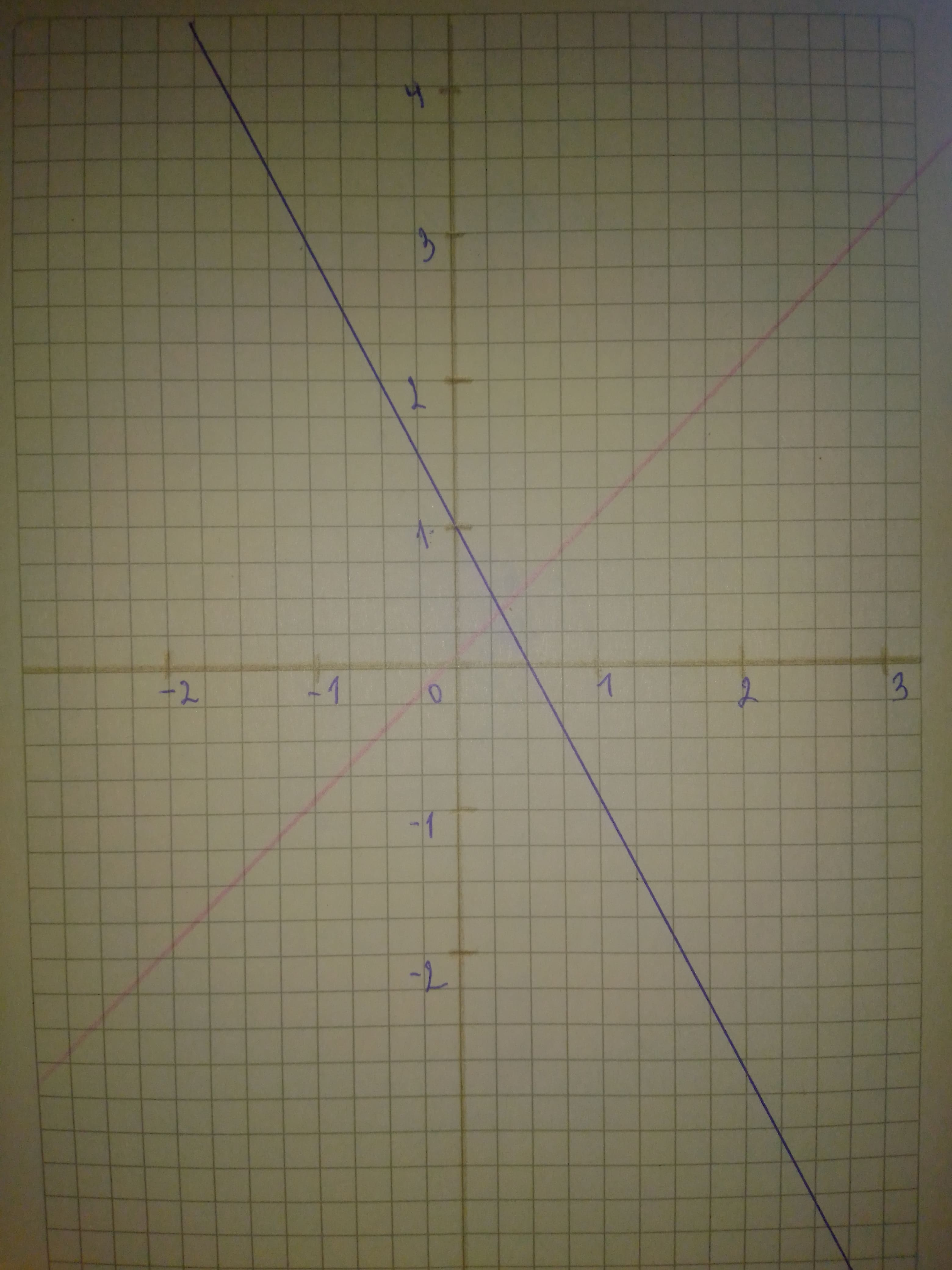# Sketch a graph of the function. Use transformations of functions whenever possible. f(x)=1-2xgenerals336 2020-12-25 Answered
Sketch a graph of the function. Use transformations of functions whenever possible. $f\left(x\right)=1-2x$
You can still ask an expert for help

• Questions are typically answered in as fast as 30 minutes

Solve your problem for the price of one coffee

• Math expert for every subject
• Pay only if we can solve itwornoutwomanC

Step 1
First graph function $y=x$.
Then, to obtain graph of function $f\left(x\right)=1-2x=-2x+1,$ do following transformations:
-stretch vertically by a factor of 2
-reflect with respect to x-axis
-shift 1 unit upward
On graph: Red - $y=x$
Blue - $f\left(x\right)=1-2x$
Step 2